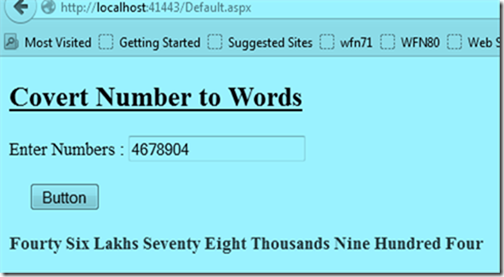Thursday, 18 December 2014

Convert number to words c# code

In many applications you need to convert numbers into words like in bank statement invoice or converting the currency values into words.
Below application will really help you to convert the numbers into words.
You just need to pass the numbers like; “23456” it will convert Twenty Three Thousands Four Hundred Fifty Six.

Application will support till the lakhs if you want to extend it you can extend till what you need.Code will be look like below;
For HTML code converting numbers to word;
<form id="form1" runat="server">
<div>
<h2><u>Covert Number to Words</u></h2>
Enter Numbers : <asp:TextBox ID="txtNumbers" runat="server"></asp:TextBox>
<br />
<br />
&nbsp;&nbsp;&nbsp;&nbsp;&nbsp;<asp:Button ID="Button1" runat="server" Text="Button" OnClick="Button1_Click" />
<br />
<br />
<asp:Label runat="server" ID="lblNumbers"  Visible="false"  ForeColor="Red" Font-Bold="true"></asp:Label>
</div>

</form>

Convert number to words c# code

protected void Page_Load(object sender, EventArgs e)
{

}
protected void Button1_Click(object sender, EventArgs e)
{
string[] DigitinOnes = { "One", "Two", "Three", "Four", "Five", "Six", "Seven", "Eight", "Nine", "Ten", "Eleven", "Twelve", "Thirteen", "Fourteen", "Fifteen", "Sixteen", "Seventeen", "Eighteen", "Ninteen" };

string[] DigitiTens = { "Ten", "Twenty", "Thirty", "Fourty", "Fifty", "Sixty", "Seventy", "Eighty", "Ninty" };

int Number = int.Parse(txtNumbers.Text);
string strWords = string.Empty;

#region calculations

/// calculates till Lakhs
if (Number >= 2000000 && Number <= 9999999)
{
int value = Number / 1000000;
strWords = strWords + DigitiTens[value - 1] + " ";
Number = Number % 1000000;
}

if (Number > 99999 && Number <= 1999999)
{
int value = Number / 100000;
strWords = strWords + DigitinOnes[value - 1] + " Lakhs ";
Number = Number % 100000;
}

/// calculates till thousand
if (Number >= 20000 && Number <= 99999)
{
int value = Number / 10000;
strWords = strWords + DigitiTens[value - 1] + " ";
Number = Number % 10000;
}

if (Number > 999 && Number <= 19999)
{
int value = Number / 1000;
strWords = strWords + DigitinOnes[value - 1] + " Thousands ";
Number = Number % 1000;
}
//// calculate till 100ds
if (Number > 99 && Number < 1000)
{
int value = Number / 100;
strWords = strWords + DigitinOnes[value - 1] + " Hundred ";
Number = Number % 100;
}

/// Calculates till tens
if (Number > 19 && Number < 100)
{
int value = Number / 10;
strWords = strWords + DigitiTens[value - 1] + " ";
Number = Number % 10;
}

if (Number > 0 && Number < 20)
{
strWords = strWords + DigitinOnes[Number - 1];
}

#endregion Till thousand

lblNumbers.Visible = true;
lblNumbers.Text = strWords;
}